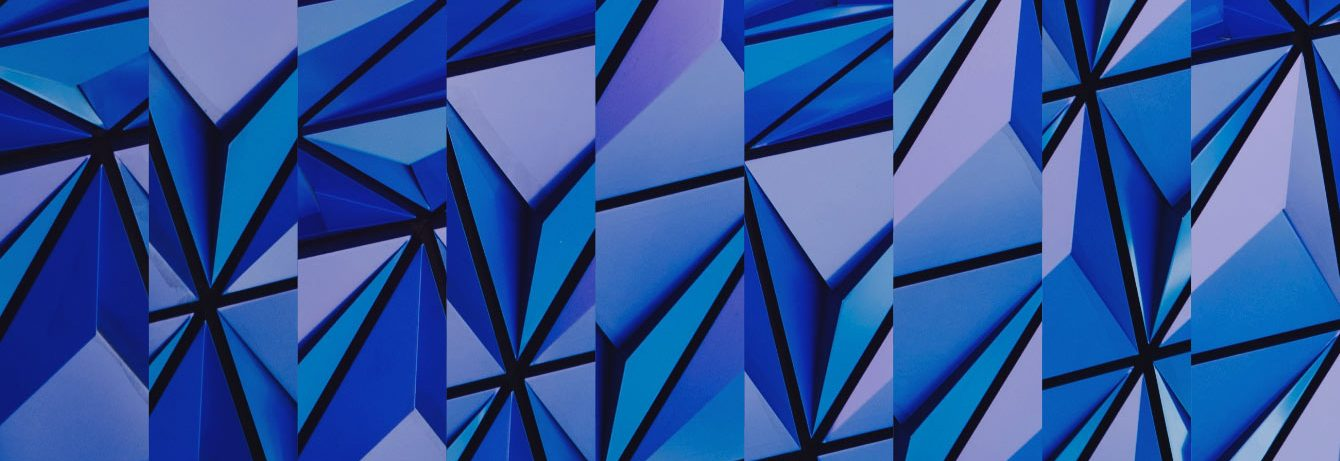GRG GroupGroups and Geometry

# Groups and Geometry (GRG)

Symmetries play a central role in mathematics.
Considering the inherent symmetries of a mathematical object allows to reduce the complexity of mathematical research problems. This often leads to an interesting interplay between geometric structures on one hand and algebraic structures on the other.
The research group “Groups and Geometry” investigates various mathematical problems in the fields of geometry and topology, which involve the interplay between geometric spaces, such as Riemannian manifolds or metric spaces, and groups, arising for example from symmetries, acting on them.

A special focus lies on the study of deformations spaces of geometric structures, moduli spaces of bundles, and Lie groups and their discrete subgroups.

Beyond our research in mathematics we are also interested in exploring possible interactions with other sciences.